RRB JE CBT 1 - Full Test 8

# RRB JE CBT 1 - Full Test 8

Test Description

## 100 Questions MCQ Test RRB JE (Railways Junior Engineer) CBT Mock Test Series | RRB JE CBT 1 - Full Test 8

RRB JE CBT 1 - Full Test 8 for Railways 2023 is part of RRB JE (Railways Junior Engineer) CBT Mock Test Series preparation. The RRB JE CBT 1 - Full Test 8 questions and answers have been prepared according to the Railways exam syllabus.The RRB JE CBT 1 - Full Test 8 MCQs are made for Railways 2023 Exam. Find important definitions, questions, notes, meanings, examples, exercises, MCQs and online tests for RRB JE CBT 1 - Full Test 8 below.
Solutions of RRB JE CBT 1 - Full Test 8 questions in English are available as part of our RRB JE (Railways Junior Engineer) CBT Mock Test Series for Railways & RRB JE CBT 1 - Full Test 8 solutions in Hindi for RRB JE (Railways Junior Engineer) CBT Mock Test Series course. Download more important topics, notes, lectures and mock test series for Railways Exam by signing up for free. Attempt RRB JE CBT 1 - Full Test 8 | 100 questions in 90 minutes | Mock test for Railways preparation | Free important questions MCQ to study RRB JE (Railways Junior Engineer) CBT Mock Test Series for Railways Exam | Download free PDF with solutions
 1 Crore+ students have signed up on EduRev. Have you?
RRB JE CBT 1 - Full Test 8 - Question 1

### How many triangles are there in the given figure?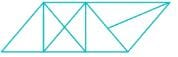Detailed Solution for RRB JE CBT 1 - Full Test 8 - Question 1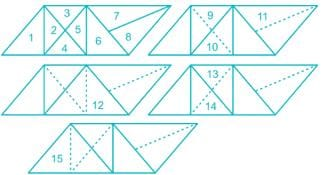Hence, the total number of triangles is 15.

RRB JE CBT 1 - Full Test 8 - Question 2

### In the following question, select the related number pair from the given alternatives. 13 : 17 :: ? : ?

Detailed Solution for RRB JE CBT 1 - Full Test 8 - Question 2

The pattern followed here is,

Consecutive prime numbers are given,

13 and 17

Similarly,

19 and 23

Hence, 23 is the correct answer.

RRB JE CBT 1 - Full Test 8 - Question 3

### In the following question, select the related word from the given alternatives. Cricket : Ball :: Badminton : ?

Detailed Solution for RRB JE CBT 1 - Full Test 8 - Question 3

The game cricket is played with bat and ball.

Similarly, badminton is played with racket and shuttle cock.

Hence Badminton is related to Shuttle.

RRB JE CBT 1 - Full Test 8 - Question 4

Three years ago, the average age of A and B was 8 years, With C joining them now, the average becomes 15 years. How old is C now?

Detailed Solution for RRB JE CBT 1 - Full Test 8 - Question 4

Let the age of A be “x” years, B be ‘y’ years and C be “z” years.

Three years ago,

Age of A = x – 3 years

Age of B = y – 3 years

Average of age of A and B = (A + B)/2 = 8 years

⇒ (x + y -6)/2 = 8

⇒ x + y – 6 = 16

⇒ x + y = 22

Now average of A,B and C = 15 years

⇒ (A + B + C)/3 = 15

⇒ x + y + z = 45

⇒ 22 + z = 45

⇒ z = 23 years

RRB JE CBT 1 - Full Test 8 - Question 5

Direction: Study the following information carefully and answer the questions given below:

Following are the conditions for selecting a Librarian in a well-known Institute:

The candidate must–

i) Be at least 30 years and not more than 40 years old as on 31.01.2018.
ii) Have completed a Bachelor Degree in Library & Information Science (BLIS) with at least 60% aggregate marks. Any Diploma or Certificate holders will not be considered.
iii) Have completed Masters in Library & Information Science (MLIS) with at least 55% marks. Any Diploma or Certificate holders will not be considered
iv) Have post qualification work experience of at least 4years as Librarian in any educational organization.

In the case of a candidate who fulfills all the conditions except:

a) At i) above, but if the candidate is not more than 55 years & has a work experience of 15 years & above as Librarian, his / her case is to be referred to Librarian Head.
b) At ii) above, but if the candidate has secured at least 55% in BLIS and at least 60% in MLIS, his / her case is to be referred to Deputy Librarian.

All these cases are given to you as on 31.01.2018

Q. Rahul Kumar has been working in a School as a Librarian for the past 5 years. He has completed his PG in Library& Information Science& secured 61%in BLIS.

Detailed Solution for RRB JE CBT 1 - Full Test 8 - Question 5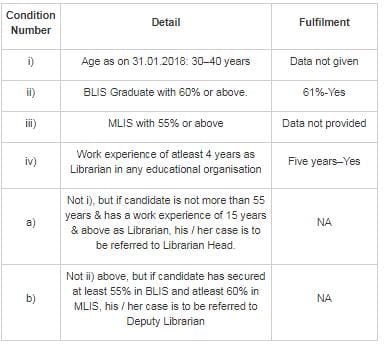Since the data about the age is not given.

RRB JE CBT 1 - Full Test 8 - Question 6

What will be the average of all the prime numbers before 19?

Detailed Solution for RRB JE CBT 1 - Full Test 8 - Question 6

Prime numbers before 19 = 17, 13, 11, 7, 5, 3, 2

We know, Average = sum of all the number/total number

∴ Average = (17 + 13 + 11 + 7 + 5 + 3 + 2)/7 = 58/7 = 8.28

RRB JE CBT 1 - Full Test 8 - Question 7

The temperature regulation center is ________

Detailed Solution for RRB JE CBT 1 - Full Test 8 - Question 7
• The hypothalamus is the processing center of the brain that controls body temperature.
• It does this by triggering changes to effectors, such as sweat glands and muscles controlling body hair.
• Heat stroke can happen when the body becomes too hot, and hypothermia when the body becomes too cold.
RRB JE CBT 1 - Full Test 8 - Question 8

Ujjain is situated on the banks of which river?

Detailed Solution for RRB JE CBT 1 - Full Test 8 - Question 8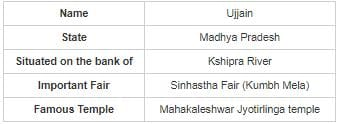RRB JE CBT 1 - Full Test 8 - Question 9

5 men can do a piece of work in 12 days and 10 women can do the same work in 15 days. 5 men and 10 women undertook the same work for Rs. 36,000. They finished the work in 5 days with the help of 15 children. What is the share of 5 children?

Detailed Solution for RRB JE CBT 1 - Full Test 8 - Question 9

Time taken by 5 men to do a piece of work = 12 days

∴ Work done by 5 men in 1 day = 1/12

Time taken by 10 women to do the same work = 15 days

∴ Work done by 10 women in 1 day = 1/15

With the help of 15 children they finished the work = 5 days

∴ Work done by (5 men + 10 women + 15 children) in 1 day = 1/5

So, work by 15 children in 1 day = 1/5 - 1/12 - 1/15 = 1/20

Time taken by 15 children to do the same work = 20 days

The ratio of their time = 12 : 15 : 20

We know, the efficiency is inversely proportional to the time

So, ratio of their efficiency = 1/12 : 1/15 : 1/20 = 5 : 4 : 3

So, 5 + 4 + 3 = 12 unit = 36,000

⇒ 1 unit = 3,000

⇒ 3 unit = 9,000

∴ Share of 15 children is 9,000

So, share of 1 child = 9,000/15 = 600

So, share of 5 children = 600 × 5 = 3,000

RRB JE CBT 1 - Full Test 8 - Question 10

The order of the increasing wavelength of the following colors will be ________

1. Orange
2. Indigo
3. Yellow
4. Violet

Detailed Solution for RRB JE CBT 1 - Full Test 8 - Question 10

The order of the increasing wavelength of the colors is:

• Violet
• Indigo
• Blue
• Green
• Yellow
• Orange
• Red

The visible light or visible spectrum is divided into these colors.

RRB JE CBT 1 - Full Test 8 - Question 11

In the following question, select the odd letter pair from the given alternatives.

Detailed Solution for RRB JE CBT 1 - Full Test 8 - Question 11

In all pairs except ‘LMNO – LMON’ 1st & 2nd and 3rd & 4th alphabets are interchanged while in ‘LMNO – LMON’ 1st & 2nd alphabets are not interchanged.

Hence, ‘LMNO – LMON’ is an odd letter pair.

RRB JE CBT 1 - Full Test 8 - Question 12

Which of the following deals with the study of Fungi?

Detailed Solution for RRB JE CBT 1 - Full Test 8 - Question 12

Mycology is the branch of biology concerned with the study of fungi, including their genetic and biochemical properties, their taxonomy and their use to humans as a source for tinder, medicine, wine, cheese, (edible mushrooms), and entheogens, as well as their dangers, such as poisoning or infection.

RRB JE CBT 1 - Full Test 8 - Question 13

What is used to prevent freezing of fuel in space crafts?

Detailed Solution for RRB JE CBT 1 - Full Test 8 - Question 13

In space craft freezing of fuel is prevented by the Usage of Glycol. It is an additive which lowers the freezing point of a water-based liquid and increases its boiling point. Thus ensuring performance of the engine or the space craft in situation of extreme temperature shifts.

RRB JE CBT 1 - Full Test 8 - Question 14

Chandragupta Maurya died in which among the following places?

Detailed Solution for RRB JE CBT 1 - Full Test 8 - Question 14
• Chandragupta Maurya became a Jain and left for Sravanbelgola, Karnataka along with his Jain guru Bhadrabahu.
• He died by slow starvation at Chandragiri Hill, Sravanbelgola .
• He lived as an ascetic for some years and died of voluntary starvation.
• He was the founder of the Maurya Dynasty in India.
RRB JE CBT 1 - Full Test 8 - Question 15

Where is 'Lippa Asrang' Wildlife Sanctuary situated?

Detailed Solution for RRB JE CBT 1 - Full Test 8 - Question 15
• 'Lippa Asrang' Wildlife Sanctuary is a part of district Kinnaur in Himachal Pradesh.
• It is situated in an area of 3089 hectares and is part of Moorang town.
• The sanctuary was established in the year 1974.
RRB JE CBT 1 - Full Test 8 - Question 16

What is the value of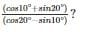Detailed Solution for RRB JE CBT 1 - Full Test 8 - Question 16

Concept:

cos(90−θ)=sinθ
sin(90−θ)=cosθ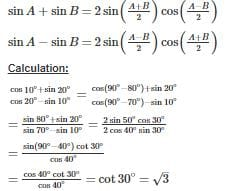RRB JE CBT 1 - Full Test 8 - Question 17

What is the constituent of tear gas?

Detailed Solution for RRB JE CBT 1 - Full Test 8 - Question 17

Tear gas also known as lachrymator agent is a chemical compound that is mostly used as a weapon as it causes severe eye, respiratory and skin irritation.

Chloropicrin is a constituent of tear gas and is responsible for causing irritation.

RRB JE CBT 1 - Full Test 8 - Question 18

Water in an electric kettle becomes hot by ________.

Detailed Solution for RRB JE CBT 1 - Full Test 8 - Question 18
• Water in an electric kettle becomes hot by convection. It is the process of transfer of heat by mass motion of a fluid such as water when the heated fluid is caused to move away from the source of it and carrying energy with it.
• Conduction is the process of transmission of heat through the material of a substance.
• Radiation is the process of emission of energy in the form of moving subatomic particles or electromagnetic waves.
RRB JE CBT 1 - Full Test 8 - Question 19

In the following figure, rectangle represents Animators, circle represents Dancers, triangle represents Potters and square represents Mothers. Which set of letters represents Potters who are not Mothers?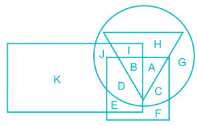Detailed Solution for RRB JE CBT 1 - Full Test 8 - Question 19

Rectangle represents Animators,

Circle represents Dancers,

Triangle represents Potters,

Square represents Mothers

Thus the letters inside the triangle but not in the intersection of square and triangle represent Potters who are not Mothers i.e. IH.

Hence, the correct answer is IH.

RRB JE CBT 1 - Full Test 8 - Question 20

M is the largest three-digit number which when divided by 6 and 5 leaves remainder 5 and 3 respectively. What will be the remainder when M is divided by 11?

Detailed Solution for RRB JE CBT 1 - Full Test 8 - Question 20

Lcm of (6, 5) = 30

Let’s assume the number be "30K + constant", where Constant is the remainder.

let that number be ‘M’

⇒ M/6 = 5 (remainder)

M could be 5, 11, 17, 23, 29, ...

⇒ M/5 = 3 (remainder)

M could be 3, 8, 13, 18, 23, 28, ...

The very first number common in both term is 23.

⇒ M is 23 i.e. a constant term

⇒ 30K + constant = 30K + 23

The largest three digit number comes when K is 32

⇒ 30 (32) + 23 = 983

∴ When 983 is divided by 11, leaves the remainder 4.

RRB JE CBT 1 - Full Test 8 - Question 21

Which element is generally NOT radioactive?

Detailed Solution for RRB JE CBT 1 - Full Test 8 - Question 21
• A radioactive element is that which breaks down over the time by turning into different elements and releasing energy.
• They are considered unstable since they have an unbalanced number of protons and neutrons in the nucleus. Germanium is not a radioactive element, while the rest of these are radioactive.
RRB JE CBT 1 - Full Test 8 - Question 22

Harike wetland is situated on the confluence of

Detailed Solution for RRB JE CBT 1 - Full Test 8 - Question 22

Harike Wetland is a manmade lake and wetland formed by the headworks built in 1953 on the confluence of the Beas and the Sutlej rivers. The wetland is characterized by the Harike freshwater lake and is a home to over 200 species of birds along with a rich marine flora and fauna.

RRB JE CBT 1 - Full Test 8 - Question 23

In the following question, select the number which can be placed at the sign of question mark (?) from the given alternatives.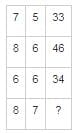Detailed Solution for RRB JE CBT 1 - Full Test 8 - Question 23

Consider the horizontal relations,

In row 1, (7 × 5) – 2 = 35 – 2 = 33

In row 2, (8 × 6) – 2 = 48 – 2 = 46,

In row 3, (6 × 6) – 2 = 36 – 2 = 34,

Similarly in row 4, (8 × 7) – 2 = 56 – 2 = 54

Hence, 54 is the correct alternative.

RRB JE CBT 1 - Full Test 8 - Question 24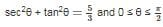then the value of θ -

Detailed Solution for RRB JE CBT 1 - Full Test 8 - Question 24

sec2θ + tan2θ =53=53

[1 + tan2θ = sec2θ]

1 + tan2θ + tan2θ =53=53

2 tan2θ  = 5/3−1

tanθ =1/√3

∴ θ = 30°

RRB JE CBT 1 - Full Test 8 - Question 25

A dishonest shopkeeper uses a weight of 850 gm instead of 1 kg and sells rice on its cost price. What will be his profit percentage on selling 5 kg rice?

Detailed Solution for RRB JE CBT 1 - Full Test 8 - Question 25

Original weight of rice = 1 kg = 1000 gm

Let cost of 1 gm is Rs. 1

Cost price of 1000 gm = Rs. 1000

Selling price of 850 gm = Rs. 1000 (∵ giving only 850 gm instead of 1000 gm)

Profit = [error/ (true weight)] × 100

⇒ Profit = [(1000 - 850)/ 850] × 100 = (150/850) × 100 = 17.65%

∴ profit % on 5kg is equal to profit on 1 kg which is 17.65%

RRB JE CBT 1 - Full Test 8 - Question 26

Some equations have been solved on the basis of a certain system. Find the correct answer for the unsolved equation on that basis.
If 95 + 65 + 23 = 81; 56 + 23 + 62 = 48 then 56 + 26 + 32 = ?

Detailed Solution for RRB JE CBT 1 - Full Test 8 - Question 26

The pattern followed is:

95 + 65 + 23 = 81

9 × 5 + 6 × 5 + 2 × 3 → 45 + 30 + 6 = 81

Similarly;

56 + 23 + 62 = 48

5 × 6 + 2 × 3 + 6 × 2 → 30 + 6 + 12 = 48

Similarly;

56 + 26 + 32 = ?

5 × 6 + 2 × 6 + 3 × 2 → 30 + 12 + 6 = 48

Hence, 48 is the correct answer.

RRB JE CBT 1 - Full Test 8 - Question 27

The product of HCF and LCM of two numbers is 2535. The smaller number is 60% of the larger number. Find the difference of between the numbers.

Detailed Solution for RRB JE CBT 1 - Full Test 8 - Question 27

Let the first number be 5a.

So, the second number = 60% of 5a = 3/5 × 5a = 3a

HCF × LCM = 1st no. × 2nd no.

⇒ 2535 = 5a × 3a

⇒ 2535 = 15a2

⇒ a= 169

⇒ a = 13

∴ Difference between the numbers = 5a - 3a = 2a = 2 × 13 = 26

RRB JE CBT 1 - Full Test 8 - Question 28

By which process water comes out of leaves in the form of vapor?

Detailed Solution for RRB JE CBT 1 - Full Test 8 - Question 28
• Water comes out of leaves in the form of vapour by the process of transpiration.
• Evaporation occurs on the surface of a liquid when it changes into gaseous phase before reaching its boiling point.
• Condensation is the change of the physical state of matter from gas to liquid.
• Sublimation is the direct transition of a substance from solid to the gas phase.
RRB JE CBT 1 - Full Test 8 - Question 29

The Protozoa which is responsible for dysentery is ______

Detailed Solution for RRB JE CBT 1 - Full Test 8 - Question 29
• Dysentery is a disease which affect the small intestine of a human being.
• It includes the severe diarrhea and abdominal pain.
• It is caused by a parasite which is known as Entamoeba histolytica.
• It is an inflammatory disease which causes inflammation in the small intestine.

RRB JE CBT 1 - Full Test 8 - Question 30

Which of the following is not a programming language?

Detailed Solution for RRB JE CBT 1 - Full Test 8 - Question 30

Linux is an operating system, not a programming language. It is a free & open-source operating system.

RRB JE CBT 1 - Full Test 8 - Question 31

Arrange the functions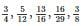In their ascending order of magnitude.

Detailed Solution for RRB JE CBT 1 - Full Test 8 - Question 31

Converting fractions to decimals,

⇒ 3/4 = 0.75

⇒ 5/12 = 0.4167

⇒ 13/16 = 0.8125

⇒ 16/29 = 0.5517

⇒ 3/8 = 0.375

∵ 0.375 < 0.4167 < 0.5517 < 0.75 < 0.8125

∴ 3/8 < 5/12 < 16/29 < 3/4 < 13/16

RRB JE CBT 1 - Full Test 8 - Question 32

If a mirror is placed on the line MN, then which of the answer figures is the right image of the given figure?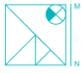Detailed Solution for RRB JE CBT 1 - Full Test 8 - Question 32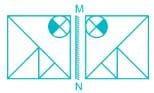Hence, option 3) is the correct mirror image of the question figure.

RRB JE CBT 1 - Full Test 8 - Question 33

Each of the following question and two statements numbered I and II given below it. You have to decide whether the data give in the statements are sufficient to answer the question. Read both the statements and choose the most appropriate answer.

How is Azad related to Shifa?
I) Shifa is the daughter of Taimur.
II) Azad is the child of Amreen, who is the wife of Taimur.

Detailed Solution for RRB JE CBT 1 - Full Test 8 - Question 33

I) Shifa is the daughter of Taimur.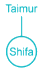We can’t determine relation between Shifa with Azad as Azad is not mentioned here.

II) Azad is the child of Amreen, who is the wife of Taimur.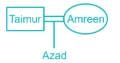We can’t determine relation between Shifa with Azad as Shifa is not mentioned here.

But, when combined together, we get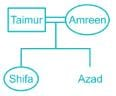As the gender of Azad is not determined, we cannot say whether he is the sister or brother of Shifa.

Hence, the data even in the both statements I and II together are not sufficient to answer the question.

RRB JE CBT 1 - Full Test 8 - Question 34

The GST on Haj has been reduced from 18 percent to ________ percent in January 2019.

Detailed Solution for RRB JE CBT 1 - Full Test 8 - Question 34
• Union Minister for Minority Affairs Mukhtar Abbas Naqvi announced that the GST on Haj has been reduced from 18 per cent to 5 per cent.
• The move will save Haj pilgrims’ Rs 113 crore this year by lowering the airfare.
• He also inaugurated a new conference hall and passport section at the Haj House in Mumbai.
RRB JE CBT 1 - Full Test 8 - Question 35

French established their ﬁrst factory at Surat and second factory at ________.

Detailed Solution for RRB JE CBT 1 - Full Test 8 - Question 35

• Under the command of Francois Curan, the French East India Company established the first French factory in India at Surat in 1668.

• The second factory was established by Marcara in 1669 at Masulipatnam

RRB JE CBT 1 - Full Test 8 - Question 36

P can do a piece of work in 20 days and Q can do the same piece of work in 60 days. They start working together and work for 5 days and then both leave the work. R alone ﬁnishes the remaining work in 10 days. In how many days will R alone ﬁnish the whole work?

Detailed Solution for RRB JE CBT 1 - Full Test 8 - Question 36

Work done by P in 1 day = 1/20

Work done by Q in 1 day = 1/60

Work done P and Q in 1 day = 1/20 + 1/60 = 1/15

Work done by P and Q in 5 days = 5 ⨯ 1/15 = 1/3

∴ P and Q finished 1/3rd of the work in 5 days.

R finished 2/3rd of the work in 10 days

2/3 work = 10 days

1 work = 10 × (3/2) = 15 days

∴ R will finish the whole work in 15 days.

RRB JE CBT 1 - Full Test 8 - Question 37

In the following question, four groups of three numbers are given. In each group, the second and third number is related to the first number by a Logic/Rule/Relation. Three are similar on the basis of the same Logic/Rule/Relation. Select the odd one out from the given alternatives.

Detailed Solution for RRB JE CBT 1 - Full Test 8 - Question 37

The patter followed is,

1) (2, 3, 4): 2 + 1 = 3; 3 + 1 = 4;

2) (4, 5, 6): 4 + 1 = 5; 5 + 1 = 6;

3) (6, 7, 8): 6 + 1 = 7; 7 + 1 = 8;

4) (4, 6, 8): 4 + 2 = 6; 6 + 2 = 8

Hence, (4, 6, 8) is the correct answer.

RRB JE CBT 1 - Full Test 8 - Question 38

Direction: Arrange the given words in reverse order in which they occur in the dictionary and choose the one that comes third after arranging in reverse order.
Grieve, Graze, Grass, Grief

Detailed Solution for RRB JE CBT 1 - Full Test 8 - Question 38

On arranging in reverse word as per dictionary order,

i) Grieve

ii) Grief

iii) Graze

iv) Grass

Hence, “Graze” comes third after arranging in reverse word as per dictionary order.

RRB JE CBT 1 - Full Test 8 - Question 39

The sharp pain caused by the sting of an ant is due to ________.

Detailed Solution for RRB JE CBT 1 - Full Test 8 - Question 39
• Ant bite injects formic acid that will irritate our nerves and triggers an inflammatory response.
• So, the sharp pain caused by the sting of an ant is due to formic acid.
RRB JE CBT 1 - Full Test 8 - Question 40

A man sells an article at 10% above its cost price. If he had bought it at 10% less than what he paid for it and sold it at Rs 11 less, he would have gained 10%. The cost price of the article is∶

Detailed Solution for RRB JE CBT 1 - Full Test 8 - Question 40

Let the cost price of the article be 100 unit

So, SP of the article = 100 × 110/100 = 110 unit

If he had bought it at 10% less, then new CP of the article = 100 × 90/100 = 90 unit

If he had paid for the article Rs. 11 less, he would have gained 10%

According to the question

⇒ (90 × 110/100) unit = 110 unit – 11

⇒ (110 – 99) unit = 11

⇒ 11 unit = 11

⇒ 100 unit = 100

∴ Cost price of the article Rs. 100

RRB JE CBT 1 - Full Test 8 - Question 41

Directions: The question consists of two statements, an assertion (A) and a reason (R). Read both the statements and decide which of the following answer choices correctly depicts the relationship between these two statements.

A: Marie Curie is the only woman to have won the Nobel Prize in Physics and Chemistry.
R: Her contribution to the world of Physics and Chemistry was incredible.

Detailed Solution for RRB JE CBT 1 - Full Test 8 - Question 41

The correct answer is option 1. Both A and R are true and R is the correct explanation for A.

Marie Curie won two Nobel prizes. One for chemistry and the other for physics. One doesn't win Nobel prize easily. Her contribution was remarkable which is why she won.

RRB JE CBT 1 - Full Test 8 - Question 42

Outer coating of a capsule is made up of ________.

Detailed Solution for RRB JE CBT 1 - Full Test 8 - Question 42
• The outer coating of a capsule is made up of gelatin.
• The traditional capsules are made from gelatin, while the soft-shelled capsules are made of liquids or oils.
• Gelatin is an animal protein which is flavorless, colorless, brittle, and translucent food ingredient derived from collagen.
• It is commonly used in medications, vitamin capsules, medications, drugs, cosmetics, gelling agent in food, etc.
RRB JE CBT 1 - Full Test 8 - Question 43

If '98' is coded as 'IH', '35' is coded as 'CE', then how will '165' is coded?

Detailed Solution for RRB JE CBT 1 - Full Test 8 - Question 43

Alphabets are coded as per their place value

98 → IH

35 → CE

Similarly,

165 → AFE

Hence, '165' is coded as 'AFE'

RRB JE CBT 1 - Full Test 8 - Question 44

Newton’s second law of motion gives us the magnitude of ________.

Detailed Solution for RRB JE CBT 1 - Full Test 8 - Question 44
• According to Newton’s second law, Force acting on a body is equal to the product of the mass and acceleration of that body, assuming a constant mass.
• This law also states that Force is equal to the change in momentum per change in time.
RRB JE CBT 1 - Full Test 8 - Question 45

If the amount received at the end of 2nd and 3rd year at Compound Interest on a certain Principal is Rs 1,800, and Rs 1,926 respectively, what is the rate of interest?

Detailed Solution for RRB JE CBT 1 - Full Test 8 - Question 45

Let the principal be P

⇒ P(1 + r/100)2 = 1800

⇒ 1 + r/100 = √(1800/P)      ----(i)

Also, P(1 + r/100)3 = 1926

⇒ 1 + r/100 = (1926/P)1/3      ----(ii)

⇒ √(1800/P) = (1926/P)1/3

⇒ P = 1572

Putting the value of P in (i), we get

⇒ 1 + r/100 = √(1800/1572)

⇒ 1 + r/100 = 1.07

⇒ r/100 = 0.07

∴ r = 7%

RRB JE CBT 1 - Full Test 8 - Question 46

What is the general formula of Alkene?

Detailed Solution for RRB JE CBT 1 - Full Test 8 - Question 46

In organic chemistry, an alkene is an unsaturated hydrocarbon that contains at least one carbon-carbon double bond. The words alkene and olefin are often used interchangeably.

The formula of Alkane - CnH(2n+2)

The formula of Alkene - CnH(2n)

The formula of Alkyne - CnH(2n-2)

RRB JE CBT 1 - Full Test 8 - Question 47

Three numbers form an increasing GP. If the middle number is doubled, then the new numbers are in AP. The common ratio of the GP is

Detailed Solution for RRB JE CBT 1 - Full Test 8 - Question 47

Let the three numbers be a/r, a, ar. As the numbers form an increasing GP. So, r > 1. It is given that a/r, 2a, ar are in A.P.

From the arithmetic mean concept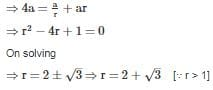RRB JE CBT 1 - Full Test 8 - Question 48

Henry is the unit of ________.

Detailed Solution for RRB JE CBT 1 - Full Test 8 - Question 48
• Henry is the unit of inductance, represented with a symbol H or L.
• Inductance, in electronics and electromagnetism, is the property of an electric conductor which causes an electromotive force to be generated with a change in the flowing current.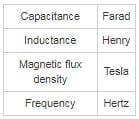RRB JE CBT 1 - Full Test 8 - Question 49

In a row of persons, the position of C from the right side of the row is 12th and position of D from the left side of the row is 18th. If G is sitting just in the middle of C and D. Find the position of G from the left side of the row.

Detailed Solution for RRB JE CBT 1 - Full Test 8 - Question 49

Since, the total no. of persons or the position of G from any of the sides is not given, the answer to the question cannot be determined.

Hence, answer is ‘Cannot be determined’.

RRB JE CBT 1 - Full Test 8 - Question 50

A statement is given followed by two inferences I and II. You have to consider the statement to be true even if it seems to be at variance from commonly known facts. You have to decide which of the given inferences, if any, follow from the given statement.

Statement:
Books without knowledge of life are useless.

Inferences:
I. All books contain the knowledge of life.
II. People should try to gain the knowledge of life.

Detailed Solution for RRB JE CBT 1 - Full Test 8 - Question 50

The statement asserts that books should contain the knowledge of life. It cannot be inferred whether it is true for all books. Thus inference I does not follow. Inference II also does not follow because the statement does not relate to people in any way.

RRB JE CBT 1 - Full Test 8 - Question 51

A series is given with one term missing. Select the correct alternative from the given ones that will complete the series
A5H, F15J, K25L, P35N, ?

Detailed Solution for RRB JE CBT 1 - Full Test 8 - Question 51

Given Series: A5H, F15J, K25L, P35N, ?

Logic Followed here is,

⇒ A + 5 = F; F + 5 = K; K + 5 = P; P + 5 = U

⇒ 5; 5 + 10 = 15; 15 + 10 = 25; 25 + 10 = 35; 35 + 10 = 45

⇒ H + 2 = J; J + 2 = L; L + 2 = N; N + 2 = P

Hence, U45P will be the next term.

RRB JE CBT 1 - Full Test 8 - Question 52

When is the World Wetlands Day celebrated?

Detailed Solution for RRB JE CBT 1 - Full Test 8 - Question 52
• World Wetlands Day is celebrated every year on 2 February.
• It marks the anniversary of the signing of the Convention on Wetlands of International Importance (Ramsar Convention) in Ramsar, Iran, on 2 February 1971.
• The international theme for World Wetlands Day 2019 is 'Wetlands and climate change'
• World Wetlands Day was first celebrated in 1997.
RRB JE CBT 1 - Full Test 8 - Question 53

A and B together have six times the sum what B and C together have, while A, B, and C together have Rs. 30 more than the sum that A has. If B has four times the sum than that of C, the sum A has, is approximate –

Detailed Solution for RRB JE CBT 1 - Full Test 8 - Question 53

Given,

A and B together have six times the sum what B and C together have

∴ A + B = 6(B + C)…..(1)

A, B and C together have Rs. 30 more than the sum that A has

∴ A + B + C = A + 30

⇒ B + C = 30…..(2)

B has four times the sum than that of C.

∴ B = 4C…..(3)

Using (2) and (3)

4C + C = 30

⇒ 5C = 30

⇒ C = Rs. 6

Putting this in (2)

B + 6 = 30

⇒ B = Rs. 24

Putting value of B and C in (1)

A + 24 = 6(24 + 6)

⇒ A = 180 – 24 = Rs. 156

RRB JE CBT 1 - Full Test 8 - Question 54

The oil used in the flotation method for the purification of ores is ________.

Detailed Solution for RRB JE CBT 1 - Full Test 8 - Question 54
• The oil used in the flotation method for the purification of ores is pine oil.
• It is used in froth flotation process since it attracts impurities which can be washed away and it does not have any affinity towards water.
• it is an essential oil which is obtained by steam distillation of twigs, cones, and needles from different species of pines.
• The process of selectively separating hydrophobic materials from hydrophilic is known as froth flotation.
RRB JE CBT 1 - Full Test 8 - Question 55

Which of the following potassium compounds is known as 'Pearl ash'?

Detailed Solution for RRB JE CBT 1 - Full Test 8 - Question 55
• K2CO3 or potassium carbonate is known as pearl ash.
• Pearl ash, in ancient times, was created by baking potash in a kiln in order to remove impurities. The remaining fine, white powder was pearl ash.
• Potassium carbonate is an inorganic compound and a white salt which is soluble in water.
• It is mainly used in the production of glass and soap.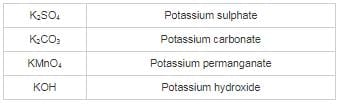RRB JE CBT 1 - Full Test 8 - Question 56

Find the ratio of third proportional to 12 and 30 and fourth proportional to 11, 15 and 33.

Detailed Solution for RRB JE CBT 1 - Full Test 8 - Question 56

We know that,

Third proportional to a and b = b2/a

∴ Third proportional to 12 and 30 = (30)2/12 = 900/12 = 75

We also know that,

Fourth proportional to a, b, c is given by = (b × c)/a

∴ Fourth proportional to 11, 15, 33 = (33 × 15)/11 = 45

∴ Ratio of Third proportional to 12 and 30 and Fourth proportional to 11, 15 and 33 = 75 : 45 = 5 : 3

RRB JE CBT 1 - Full Test 8 - Question 57

Find the wrong term in the following series.
4, 9, 25, 49, 64, 169, 289, 361

Detailed Solution for RRB JE CBT 1 - Full Test 8 - Question 57

The pattern followed here is,

All the given numbers are squares of the consecutive prime numbers,

For e.g.

4 = 22; 9 = 32; 25 = 52; 49 = 72…. so on.

But 64 = 82, and 8 is not a prime number.

Hence, 64 is the odd one out.

RRB JE CBT 1 - Full Test 8 - Question 58

If P means ‘+‘, Q means ‘×‘, R means ‘÷’ and S means ‘-‘, then the value of 22P 4 P 9 S(6P7S8R4) Q3P1S2 is

Detailed Solution for RRB JE CBT 1 - Full Test 8 - Question 58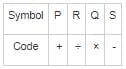Given: 22 P 4 P 9 S (6 P 7 S 8 R 4) Q 3 P 1 S 2

Substituting the symbols:

22 + 4 + 9 - (6 + 7 - 8 ÷ 4) × 3 + 1 - 2

Solution through BODMAS:

22 + 4 + 9 - (6 + 7 - 8 ÷ 4) × 3 + 1 - 2

22 + 4 + 9 - (6 + 7 - 2) × 3 + 1 - 2

22 + 4 + 9 - 11 × 3 + 1 - 2

22 + 4 + 9 - 33 + 1 - 2

22 + 4 + 9 + 1 - 33 - 2

36 - 35 = 1

Hence, the correct answer is 1

RRB JE CBT 1 - Full Test 8 - Question 59

Kyoto Protocol is associated with ________.

Detailed Solution for RRB JE CBT 1 - Full Test 8 - Question 59

The Kyoto Protocol is an international treaty which extends the 1992 United Nations Framework Convention on Climate Change (UNFCCC) that commits State Parties to reduce greenhouse gas emissions.

RRB JE CBT 1 - Full Test 8 - Question 60

On what principle, the difference between compound and simple interest shall come out to be Rs. 366, if the money is kept for 3 years at 5%?

Detailed Solution for RRB JE CBT 1 - Full Test 8 - Question 60

Let the principal = Rs. 100

Now, S.I. on Rs. 100 = PTR/100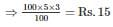Thus, S.I. on Rs. 100 = Rs. 15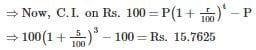⇒ C.I. on Rs. 100 = Rs. 15.7625

Now, the difference of C.I. and S.I. = 15.7625 – 15 = 0.7625

⇒ For Rs. 100 the difference in C.I. and S.I. is Rs. 0.7625

⇒ For Rs. X the difference in C.I. and S.I. is Rs. 366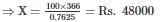∴ On Rs. 48000, the difference of compound and simple interest, shall come out to be Rs. 366

RRB JE CBT 1 - Full Test 8 - Question 61

The xylem in plants are responsible for:

Detailed Solution for RRB JE CBT 1 - Full Test 8 - Question 61

Xylem is one of the two types of transport tissue in vascular plants, phloem being the other. The basic function of xylem is to transport water from roots to shoots and leaves, but it also transports some nutrients.

RRB JE CBT 1 - Full Test 8 - Question 62

A number is increased by 18%. To obtain the old number, by how much percentage should it be decreased?

Detailed Solution for RRB JE CBT 1 - Full Test 8 - Question 62

Given,

A number is increased by 18%

Let number be n.

New number =

= n + n × (18/100)

= 59n/50

To obtain old number,

Let percentage number should be decreased to obtain new number be a.

n = 59n/50 - (59n/50) × (a/100)

n = (5900n - 59na)/5000

5000n = 5900n - 59na

59na = 900n

a = 900n/59n

a = 15.25

By 15.25% new number should be decreased to obtain new number.

RRB JE CBT 1 - Full Test 8 - Question 63

Direction: The question given below consists of two statements numbered I and II given below it. You have to decide whether the data provided in the statements are sufficient to answer the question. Read both the statements and give the answer:

In a row, there are 5 poles - P, Q, R, S, and T,  which pole is at the penultimate position?

Statements:
I. Poles S and Q are at the two extreme ends of the row respectively from left to right.
II. Pole T is to the right of building R.

Detailed Solution for RRB JE CBT 1 - Full Test 8 - Question 63

From I, we get the order: S, - , - , - , Q.

From II, we get the order: R, T

Combining I and II, we get two possible orders:

S, R, T, P, Q or S, P, R, T, Q.

Thus, either P or T is at the penultimate position.

RRB JE CBT 1 - Full Test 8 - Question 64

A train passes an electrical pole in 30 seconds and passes a platform 240 m long in 2 Minutes. Find the length of the train:

Detailed Solution for RRB JE CBT 1 - Full Test 8 - Question 64

Let the length of the train be D metres and speed of the train be S m/s

Now As per the basic formula of speed,

S = D/T

Where S = Speed, D = Distance, T = Time

As the train passes an electric pole in 30 seconds

S = D/30  ........eq(i)

As train passes a platform 240 m long in 120 seconds

S = (D + 240)/120  .....eq(ii)

from eq(i) and (ii) we get

D/30 = (D + 240)/120

∴ 4D = D + 240

∴ 3D = 240

∴ D = 80 m

RRB JE CBT 1 - Full Test 8 - Question 65

A statement is given followed by two inferences I and II. You have to consider the statement to be true even if it seems to be at variance from commonly known facts. You have to decide which of the given inferences, if any, follow from the given statement.

Statement:
City Y made it compulsory for the students to wear uniforms in colleges.

Inferences:
I. The city wants its college students more disciplined.
II. The city believes uniforms will bring in more discipline.

Detailed Solution for RRB JE CBT 1 - Full Test 8 - Question 65

Uniforms were made to maintain equality and motivate discipline. Therefore City Y imposing this rule shows that they believe that uniforms will instill discipline and that their students need more discipline. Consequently, option 3 is the correct answer.

RRB JE CBT 1 - Full Test 8 - Question 66

________ has won the award for the Best Supporting Actor at the Golden Globe Awards for 2019.

Detailed Solution for RRB JE CBT 1 - Full Test 8 - Question 66
• 'Roma' won the award for Best Foreign Language Picture.
• Spider-Man: Into the Spider-Verse won the award for the Best animated picture.
• Mahershala Ali won the award for the Best Supporting Actor.
• Regina King won the award for the Best Supporting Actress.
• Christian Bale won the award for the Best Actor – Musical or Comedy
• Olivia Colman won the award for the Best Actress – Musical or Comedy
RRB JE CBT 1 - Full Test 8 - Question 67

Plaster of Paris is made from ________.

Detailed Solution for RRB JE CBT 1 - Full Test 8 - Question 67
• Plaster of Paris is made from gypsum.
• The common name for plaster is calcium sulphate hemi hydrate which is made by heating gypsum or sulphate of lime.
• PoP gets its name from a huge amount of gypsum deposited on Montmartre Hill in Paris.
RRB JE CBT 1 - Full Test 8 - Question 68

The question consists of two statements, an assertion, and a reason. The student must first determine whether each statement is true. Each question below consists of an assertion and a reason.

Assertion (A): Mahatma Gandhi is the Father of the Nation (India).

Reason (R): Gandhi’s picture is printed on the Indian currency notes.

Detailed Solution for RRB JE CBT 1 - Full Test 8 - Question 68

It is true that Mahatma Gandhi is the Father of the Nation of India and hence, A is true. It is also true that Gandhi’s picture is printed on the Indian currency notes, so R is also true. But Gandhi is the Father of the Nation because of his contribution to the freedom struggle and only after that was his picture printed on the currency notes. Therefore, R is not the correct explanation of A and option 2 is the correct solution.

RRB JE CBT 1 - Full Test 8 - Question 69

If the supplement of an angle is (5/2) of its complement, then find the angle.

Detailed Solution for RRB JE CBT 1 - Full Test 8 - Question 69

We know, two angles whose sum is 90° are called complementary angles and two angles whose sum is 180° are called supplementary angles

Let the angle be x

According to the question,

⇒ (180° – x) = 5/2 (90° – x)

⇒ 360° – 2x = 450° – 5x

⇒ 3x = 90°

⇒ x = 30°

∴ The angle is 30°

RRB JE CBT 1 - Full Test 8 - Question 70

The sum of 11 consecutive integers is 11. What is the lowest integer?

Detailed Solution for RRB JE CBT 1 - Full Test 8 - Question 70

Let, 11 consecutive numbers are,

⇒ (x - 5), (x - 4), (x - 3), (x - 2), (x - 1), x, (x + 1), (x + 2), (x + 3), (x + 4), (x + 5)

According to problem,

⇒ (x - 5) + (x - 4) + (x - 3) + (x - 2) + (x - 1) + x + (x + 1) + (x + 2) + (x + 3) + (x + 4) + (x + 5) = 11

⇒ 11x = 11

⇒ x = 1

∴ The lowest number = 1 - 5 = -4

RRB JE CBT 1 - Full Test 8 - Question 71

If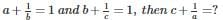Detailed Solution for RRB JE CBT 1 - Full Test 8 - Question 71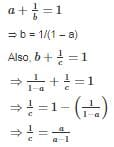⇒ c = 1 – 1/a

⇒ c + 1/a = 1

RRB JE CBT 1 - Full Test 8 - Question 72

Detailed Solution for RRB JE CBT 1 - Full Test 8 - Question 72
• Radio waves have lower frequencies and longer wavelengths as compared to the visible light waves.
• These are the kind of electromagnetic radiations having wavelengths in the electromagnetic spectrum longer than infrared light.
• They travel at the speed of light.
RRB JE CBT 1 - Full Test 8 - Question 73

Which among the following is correct regarding the removal of the Vice President from his position?

Detailed Solution for RRB JE CBT 1 - Full Test 8 - Question 73
• The Vice President can be removed from his office by a resolution of the Rajya Sabha passed by a majority of its members and agreed to by the Lok Sabha. (Article 67)
• No formal impeachment is required for the removal of the Vice President from his or her post.
• Generally, the term of the office of the Vice President is five years.
RRB JE CBT 1 - Full Test 8 - Question 74

Which one among the following is not correct about Down's Syndrome?

Detailed Solution for RRB JE CBT 1 - Full Test 8 - Question 74
• A genetic disorder which is caused by the presence of a part or all of the third copy of chromosome 21 is known as the Down's Syndrome.
• It is associated with delays in physical growth, characteristic facial features, and moderate to mild intellectual disability.
• A person affected with it is mentally retarded and has a furrowed tongue.
• The parents are typically genetically normal of the affected individual.
RRB JE CBT 1 - Full Test 8 - Question 75

If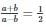then what is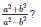Detailed Solution for RRB JE CBT 1 - Full Test 8 - Question 75

Applying componendo - dividendo,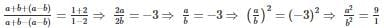Again applying componendo - dividendo,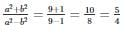RRB JE CBT 1 - Full Test 8 - Question 76

The side mirrors of vehicles are of which type of mirrors?

Detailed Solution for RRB JE CBT 1 - Full Test 8 - Question 76

The side mirrors of vehicles are convex mirrors. Because the image is smaller, more space can be covered by this mirror, so a convex mirror provides for a larger field of view than a plane mirror. This is why they are used whenever a mirror with a large field of view is needed. For example, the passenger-side rear view mirror on a car is convex.

RRB JE CBT 1 - Full Test 8 - Question 77

The plasma of the blood contains which among the following constituents?

I. Proteins
II. Salts
III. Hormones

Detailed Solution for RRB JE CBT 1 - Full Test 8 - Question 77
• Plasma is the largest component of blood, comprising about 55% of its overall content.
•  Along with water, plasma carries salts, hormones and enzymes.
• The main purpose of plasma is to transport nutrients, hormones, and proteins to the parts of the body that need it.
RRB JE CBT 1 - Full Test 8 - Question 78

The ‘Father of Indian Space Program’ is-

Detailed Solution for RRB JE CBT 1 - Full Test 8 - Question 78

Vikram Ambalal Sarabhai (12 August 1919 – 30 December 1971) was an Indian scientist and innovator widely regarded as the father of India's space programme. Sarabhai received the Shanti Swarup Bhatnagar Medal in 1962. The nation honoured him awarding Padma Bhushan in 1966 and Padma Vibhushan (posthumously) in 1972

RRB JE CBT 1 - Full Test 8 - Question 79

Uber cup associated with which sport?

Detailed Solution for RRB JE CBT 1 - Full Test 8 - Question 79
• Uber cup associated with Badminton.
• It is named after a former British women's badminton player, Betty Uber.
• It is often called World Team Championships for Women.
• It is a major international badminton competition contested by women's national badminton teams.
• Thomas Cup is the World Team Championship for Men in Badminton.
RRB JE CBT 1 - Full Test 8 - Question 80

What is the full form of WAN?

Detailed Solution for RRB JE CBT 1 - Full Test 8 - Question 80

A wide area network is a telecommunications network or computer network that extends over a large geographical distance/place. Wide area networks are often established with leased telecommunication circuits.

RRB JE CBT 1 - Full Test 8 - Question 81

Direction: Read the information given below and answer the question that follows.

Jyoti, Vipul, Disha, Ramki, Ralston, Abha, Kiran, and Anjali are sitting around a circular table facing outside.

Anjali does not sit adjacent to Ramki. Disha sits two places away to the right of Ramki. Vipul does not sit adjacent to Ramki. Ralston sits exactly between Vipul and Kiran. Vipul sits exactly between Ramki and Disha. Only one person sits between Ramki and Abha. Ralston sits opposite Anjali.

Q. Who sits opposite to Ramki?

Detailed Solution for RRB JE CBT 1 - Full Test 8 - Question 81

Names: Jyoti, Vipul, Disha, Ramki, Ralston, Abha, Kiran and Anjali

1. Disha sits two places away to the right of Ramki. (Two places away mean one person in between).

2. Only one person sits between Ramki and Abha.

3. Anjali does not sit adjacent to Ramki.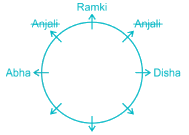4. Vipul does not sit adjacent to Ramki.

5. Vipul sits exactly between Ramki and Disha.

Note: exactly between doesn't mean persons should be the neighbors. Count from the left of Ramki and right of Disha.

B exactly between A and C means the number of persons between A and B is equal to the number of persons between B and C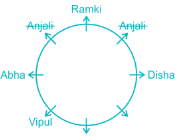6. Ralston sits exactly between Vipul and Kiran.

7. Ralston sits opposite to Anjali.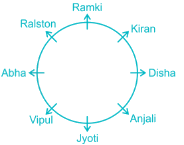Hence, Jyoti sits opposite to Ramki.

RRB JE CBT 1 - Full Test 8 - Question 82

Direction: Read the information given below and answer the question that follows.

Jyoti, Vipul, Disha, Ramki, Ralston, Abha, Kiran, and Anjali are sitting around a circular table facing outside.

Anjali does not sit adjacent to Ramki. Disha sits two places away to the right of Ramki. Vipul does not sit adjacent to Ramki. Ralston sits exactly between Vipul and Kiran. Vipul sits exactly between Ramki and Disha. Only one person sits between Ramki and Abha. Ralston sits opposite Anjali.

Q. Who sits exactly between Anjali and Ralston?

Detailed Solution for RRB JE CBT 1 - Full Test 8 - Question 82

Names: Jyoti, Vipul, Disha, Ramki, Ralston, Abha, Kiran and Anjali

1. Disha sits two places away to the right of Ramki. (Two places away mean one person in between).

2. Only one person sits between Ramki and Abha.

3. Anjali does not sit adjacent to Ramki.4. Vipul does not sit adjacent to Ramki.

5. Vipul sits exactly between Ramki and Disha.

Note: exactly between doesn't mean persons should be the neighbors. Count from the left of Ramki and right of Disha.

B exactly between A and C means the number of persons between A and B is equal to the number of persons between B and C6. Ralston sits exactly between Vipul and Kiran.

7. Ralston sits opposite to Anjali.Thus, Kiran and Vipul is the correct answer.

RRB JE CBT 1 - Full Test 8 - Question 83

The filament of electric bulbs is generally made of ______.

Detailed Solution for RRB JE CBT 1 - Full Test 8 - Question 83
• Filament of an electric bulb is generally made of Tungsten.
• These bulbs create light by passing a current through a tungsten filament to heat it to 2700C.
• The inert gas filled in the bulb prevents the filament from oxidizing and evaporating.
• Argon is filled in electric bulbs
RRB JE CBT 1 - Full Test 8 - Question 84

If a + b + c = 8, a2 + b+ c= 30, and a3 + b+ c= 134, then the value of (abc)-1 is:

Detailed Solution for RRB JE CBT 1 - Full Test 8 - Question 84

Given, a + b + c = 8, a2 + b2 + c2 = 30 and a3 + b3 + c3 = 134

∵ (a + b + c)2 = a2 + b+ c2 + 2(ab + bc + ca)

⇒ 82 = 30 + 2(ab + bc + ca)

⇒ 2(ab + bc + ca) = 64 - 30

⇒ (ab + bc + ca) = 34/2 = 17

We know that,

∵ a3 + b+ c3 – 3abc = (a + b + c) (a2 + b+ c2 – (ab + bc + ca))

⇒ 134 – 3abc = 8 × (30 – 17) = 104

⇒ 3abc = 134 - 104 = 30

⇒ abc = 30/3 = 10

∴ (abc)-1 = 1/10

RRB JE CBT 1 - Full Test 8 - Question 85

The force acting between two electric charges is related to ________.

Detailed Solution for RRB JE CBT 1 - Full Test 8 - Question 85
• The force acting between two electric charges is related to Coulomb's law.
• Coulomb's law states that the electrostatic force of repulsion or attraction between two point charges is directly proportional to the product of charges.
• Ampere's law-  The magnetic field created by an electric current is proportional to the size of that electric current with a constant of proportionality equal to the permeability of free space.

1. Emf is induced whenever a conductor is rotated in the magnetic field.
2. The induced emf equals the rate of change of flux

• Ohm's law- The direct current flowing in a conductor is directly proportional to the potential difference between its ends.
RRB JE CBT 1 - Full Test 8 - Question 86

The income of a person is Rs. 45000 and his expenditure is Rs. 33000. If his income is increased by 22% and expenditure increased by 11%, then what will be the percentage increase in his savings?

Detailed Solution for RRB JE CBT 1 - Full Test 8 - Question 86

Initial income = Rs. 45000

Initial Expenditure = Rs. 33000

Savings = Rs. 45000 - Rs. 33000 = Rs. 12000

New Income = 45000 + 22% of 45000 = Rs. 54900

New Expenditure = 33000 + 11% of 33000 = Rs. 36630

New savings = 18270

∴ Percentage increase in savings = [(18270 - 12000)/12000] × 100 = 52.25%

RRB JE CBT 1 - Full Test 8 - Question 87

A boat goes 20 km against the stream and 32 km along the stream in 8 hours. It also goes 32 km against the stream and 20 km along the stream in 8.5 hours. What is the rate of the stream?

Detailed Solution for RRB JE CBT 1 - Full Test 8 - Question 87

Let the rate of upstream be x kmph and rate of downstream be y kmph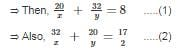Adding eq (1) and eq(2), we get

⇒ 52(1/x + 1/y) = 33/2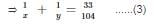Subtracting eq (1) from eq(2), we get

⇒ 12(1/x – 1/y) = 1/2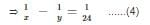Adding eq (3) and eq (4), we get

⇒ 2/x = 14/39

⇒ x = 5.57 kmph

⇒ Substitute x in eq (3), we get

⇒ 1/(5.57) – 1/y = 1/24

⇒ y = 7.25 kmph

Upstreamrate is 5.57 kmph and rate downstream is 7.25 kmph

∴ Rate of current =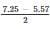= 0.84 kmph

RRB JE CBT 1 - Full Test 8 - Question 88

Two trees are standing along the opposite sides of a road. Distance between the two trees is 400 metres. There is a point on the road between the trees. The angles of depression of the point from the top of the trees are 45° and 60°. If the height of the tree which makes 45° angle is 200 metres, then what will be the height (in metres) of the other tree?

Detailed Solution for RRB JE CBT 1 - Full Test 8 - Question 88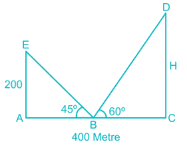In the figure, H is the height of the other tree.

In triangle AEB, AB = 200/tan45°

⇒ AB = 200 mtr

⇒ BC = 400 - 200 = 200 mtr

In triangle DCB, tan 60° = H/BC

⇒ H = 200√3 mtr

∴ Height (in metres) of the other tree = 200√3 mtr

RRB JE CBT 1 - Full Test 8 - Question 89

A mirage is formed because of which of the following phenomenon?

Detailed Solution for RRB JE CBT 1 - Full Test 8 - Question 89
• The mirage is formed because of Total Internal Reflection and refraction. It is a naturally occurring optical illusion in which light rays bend to produce a displaced image of distant objects or the sky.
• Refraction-  the change in direction of a wave passing from one medium to another or from a gradual change in the medium
• Dispersion- the separation of white light into colors or of any radiation according to the wavelength
RRB JE CBT 1 - Full Test 8 - Question 90

Akash purchased an article at a discount of 40% and sold it to Ram at 40% profit. What is the ratio between the market price and the price for which he sold?

Detailed Solution for RRB JE CBT 1 - Full Test 8 - Question 90

Let, marked price = Rs. x

Discount = 40%

∴ Cost price = x - x × 40/100 = Rs. 0.6x

Profit = 40%

∴ Selling price,

⇒ 0.6x + 0.6x × 40/100

⇒ 0.84x

∴ Required Ratio,

⇒x ∶ 0.84x

⇒ 100 ∶ 84

⇒ 25 ∶ 21

RRB JE CBT 1 - Full Test 8 - Question 91

Who among the following won the BWF World Championship 2018 women’s singles title?

Detailed Solution for RRB JE CBT 1 - Full Test 8 - Question 91
• Carolina Marin won the BWF World Championship 2018 women’s singles title.
• She belongs to Spain.
• After being defeated in the finals, P.V. Sindhu settled with the silver medal.
• In the Men's Singles, Kento Momota of Japan defeated Shi Yuqi of China to win the gold medal.
• The championship was held in China.
RRB JE CBT 1 - Full Test 8 - Question 92

A batsman scores 87 runs in the 21st match of his career. His average runs per match increases by 2. What was his average before the 21st match?

Detailed Solution for RRB JE CBT 1 - Full Test 8 - Question 92

Let his average before the 21st match be x and average after the 21st match will be x + 2

His total runs before the 21st match can be given as 20x.

From the problem statement

⇒ x + 2 = (87 + 20x)/21

⇒ x = 45

∴ His average before the 21st match is 45.

RRB JE CBT 1 - Full Test 8 - Question 93

"Yakshagana" folk dance is associated to which Indian state?

Detailed Solution for RRB JE CBT 1 - Full Test 8 - Question 93
• Combines dance, music, dialogue, costume, make-up, and stage techniques with a unique style and form. This theatre style is mainly found in all parts of Karnataka.
• Yakshagana is traditionally presented from dusk to dawn.
RRB JE CBT 1 - Full Test 8 - Question 94

A circle and a rectangle have the same perimeter. The sides of the rectangle are 18 cm and 34cm. The approximate area of the circle is :

Detailed Solution for RRB JE CBT 1 - Full Test 8 - Question 94

Perimeter of the circle = Perimeter of the rectangle

Perimeter of the circle = 2 × (length + breadth)

Perimeter of the circle = 2 × (18 + 34) = 104 cm

2πr = 104

r = 16.5 cm

Area of the circle = π × r2 = π × 16.52 = 856 cm2

∴ The area of the circle is 856 sq.cm

RRB JE CBT 1 - Full Test 8 - Question 95

Which of the following writ is issued by a higher court to a lower court?

Detailed Solution for RRB JE CBT 1 - Full Test 8 - Question 95

There are five types of Writs -Habeas Corpus, Mandamus, Prohibition, Certiorari and Quo warranto. The writ of prohibition is meant to direct a subordinate to stop doing something that the law prohibits. It is issued by a higher court to a lower court.

RRB JE CBT 1 - Full Test 8 - Question 96

For the quadratic equation x2 -3x + 2 = 0, what is the sum of the reciprocals of its roots?

Detailed Solution for RRB JE CBT 1 - Full Test 8 - Question 96

We know that if α and β are roots of a quadratic equation then the quadratic equation is of the form

x– (α + β) x + αβ = 0

So in the question, α + β = 3 and αβ = 2

Sum of the reciprocals of roots =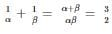RRB JE CBT 1 - Full Test 8 - Question 97

Pampas grasslands can be usually found in which of the following places?

Detailed Solution for RRB JE CBT 1 - Full Test 8 - Question 97

Pampas grasslands are fertile South American lowlands, covering more than 750, 000 km2 that include the Argentine provinces of Buenos Aires, La Pampa, Santa Fe, most of Uruguay; and the southernmost BrazilianState, Rio Grande do Sul.

RRB JE CBT 1 - Full Test 8 - Question 98

Raja is the father of Rupam, but Rupam is not his son. Pariksha is the wife of Raja. Kunal is the son of Pariksha. How is Rupam related to Pariksha?

Detailed Solution for RRB JE CBT 1 - Full Test 8 - Question 98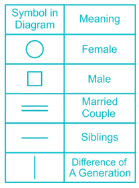The given information can be represented as follows,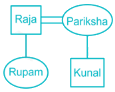Thus Rupam is the daughter of Pariksha.

RRB JE CBT 1 - Full Test 8 - Question 99

Which one of the following is a true fish?

Detailed Solution for RRB JE CBT 1 - Full Test 8 - Question 99
• A True Fish is one which goes by the strict definition of a fish having gills for respiration, fins and tail for propulsion and inner skeletal system of bones.
• Dogfish is the true fish.
RRB JE CBT 1 - Full Test 8 - Question 100

Who wrote the book “War and Peace”?

Detailed Solution for RRB JE CBT 1 - Full Test 8 - Question 100
• Russian author Leo Tolstoy is the author of the novel “War and Peace”.
• It is regarded as one of his finest works and is also a major work in the field of literature.

## RRB JE (Railways Junior Engineer) CBT Mock Test Series

19 tests
 Use Code STAYHOME200 and get INR 200 additional OFF Use Coupon Code
Information about RRB JE CBT 1 - Full Test 8 Page
In this test you can find the Exam questions for RRB JE CBT 1 - Full Test 8 solved & explained in the simplest way possible. Besides giving Questions and answers for RRB JE CBT 1 - Full Test 8, EduRev gives you an ample number of Online tests for practice

19 tests